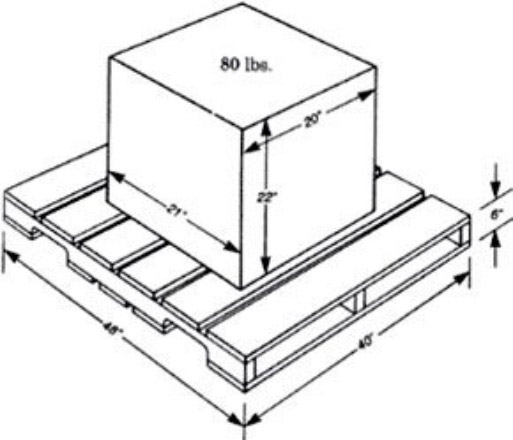To obtain the cubic dimensions of a load measured in width, length and depth, multiply the length, width and depth to obtain the dimensions in cubic inches.

Example: Length 21 “, Width 20″, Depth 22 ”
21 x 20 x 22 = 9,240 cubic inches.

To convert cubic inches into cubic feet, cubic inches are divided by 1728 (the number of one cubic inch in a cubic foot).

Example: 9.240 / 1.728 = 5.35 cubic feet

To determine density, divide the weight of the load by the number of cubic feet.

Example: 80 / 5.35 = 14.95 pounds per cubic foot
In case the load is palletized, the dimensions of the pallet determine the cubic dimensions.

Since the original dimension of the carton remains the same, the cubic dimensions now include that pallet.

Example: Length 48 “, Width 40”, Depth 28 “(22” plus 6 “of the pallet) 48 x 40 x 28 = 53,760 cubic inches.

It converts to cubic foot only by dividing between 1728.

Example: 53.760 / 1728 = 31.1 cubic feet.

Then the density is equal to the weight 110 (80 pounds per carton plus about 30 pounds per pallet) divided by the cubic dimensions.

Example: 110 / 31.1 = 3.54 pounds per cubic foot.